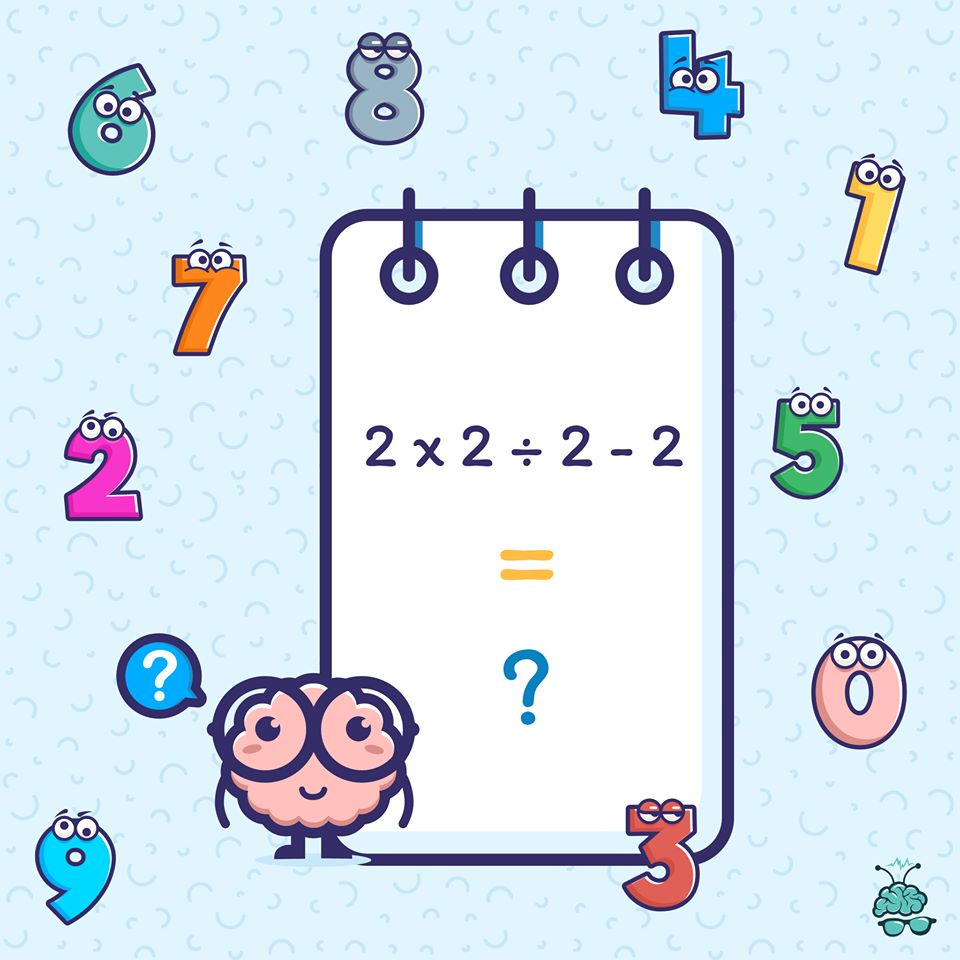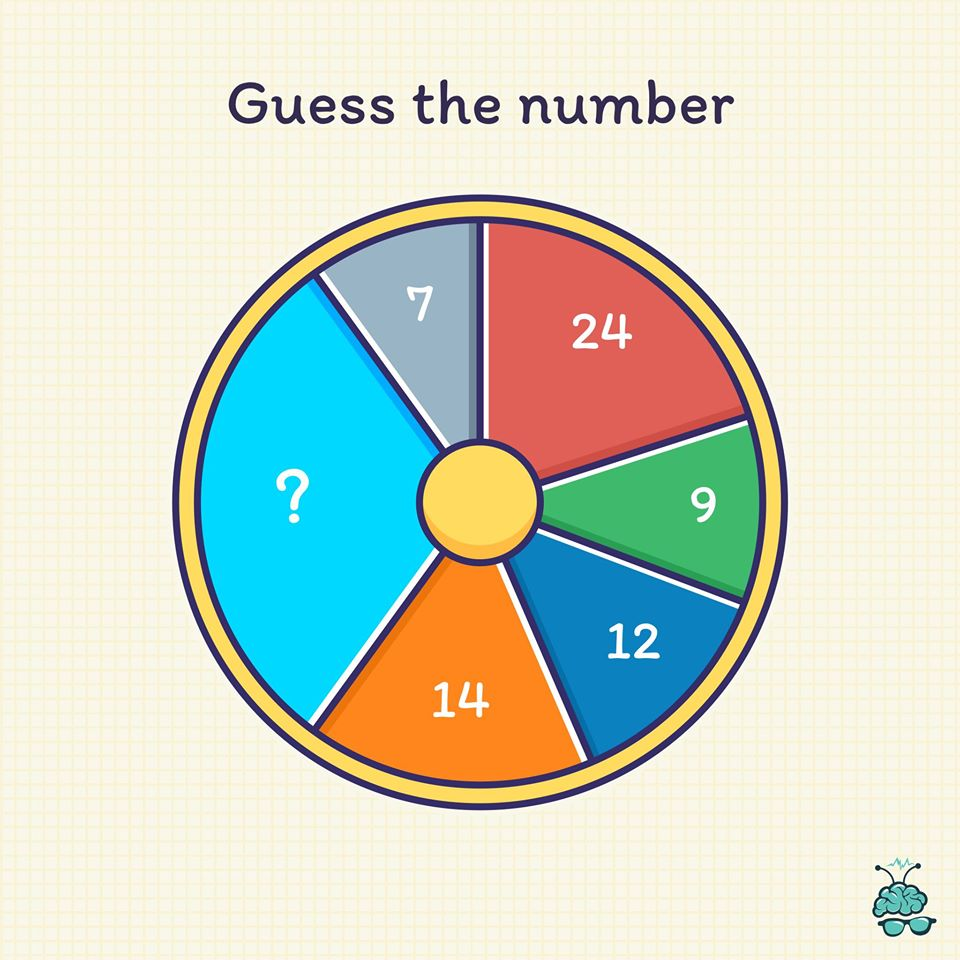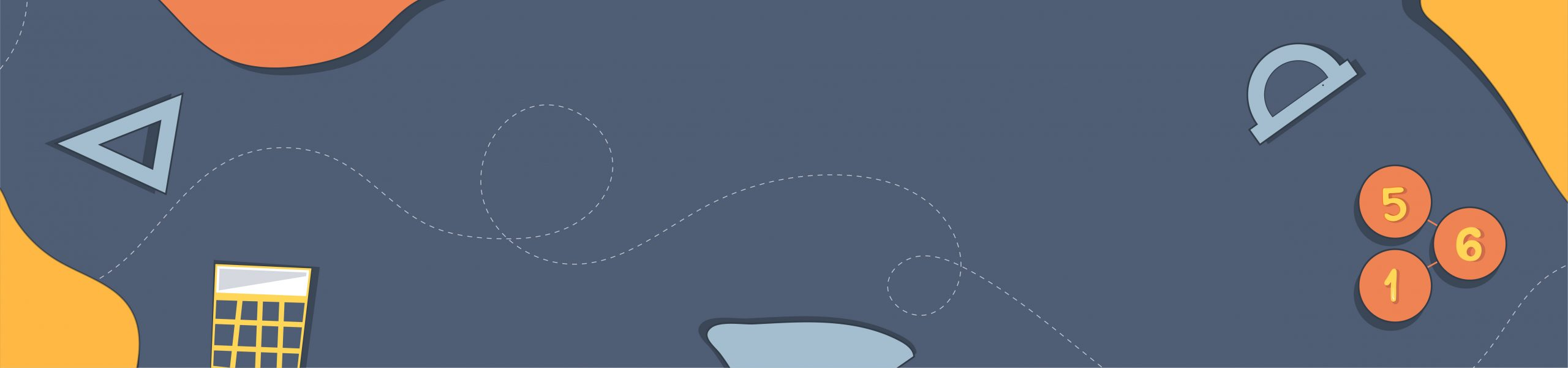# Math Puzzles

Step in the shoes of Archimedes and try to conquer these mathematical puzzles. Whether you are a math buff or someone looking to improve your problem-solving skills, our collection of unique math puzzles and number puzzles is all you need.

If you are a math lover who likes playing with the numbers and solving the math problems — these puzzles are just for you. Play around with a vast range of math puzzles and number puzzles from mental math to arithmetic, probability and algebra made for kids and adults of all ages.

## Math Puzzles, Riddles

### Nothing pumps your brain better than the old and classic puzzle problems. And today we have one for you. So can you guess the answer? Quick, share it in the comments.## Math Puzzles

### You can’t solve this brain teaser unless you’re very, very, very smart. Let’s see if you can crack this one.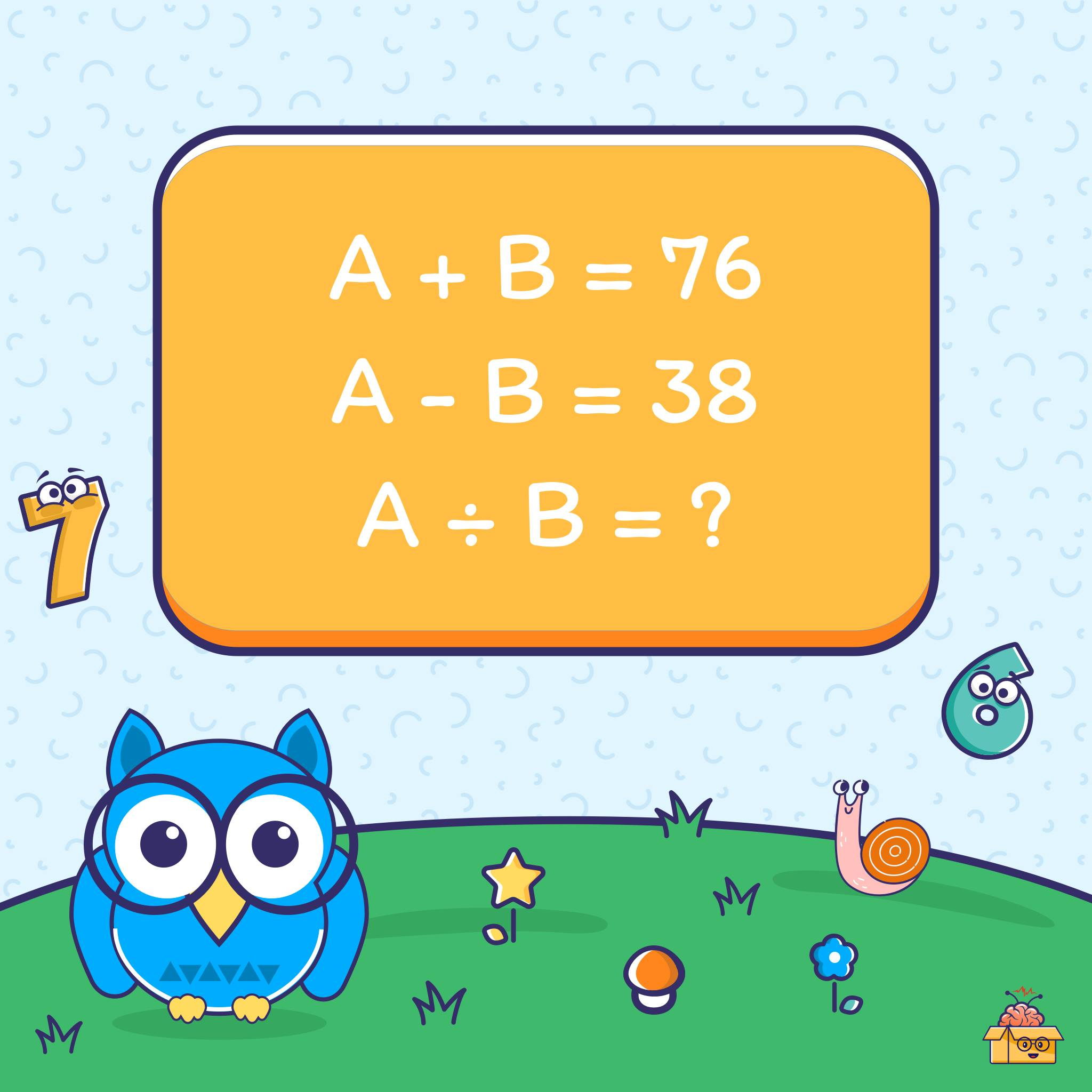## Brain Games, Math Puzzles

### Smart Brains! This is a really basic math brain teaser — but most adults can’t pass it. Can you?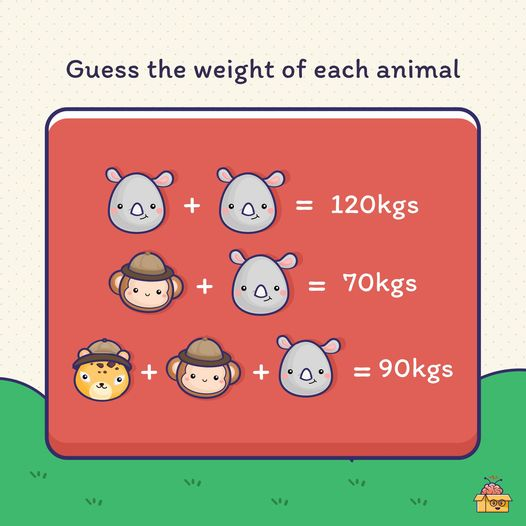## Brain Games, Math Puzzles

### Can you guess the missing number with logic? Please comment.. We are waiting for Math genius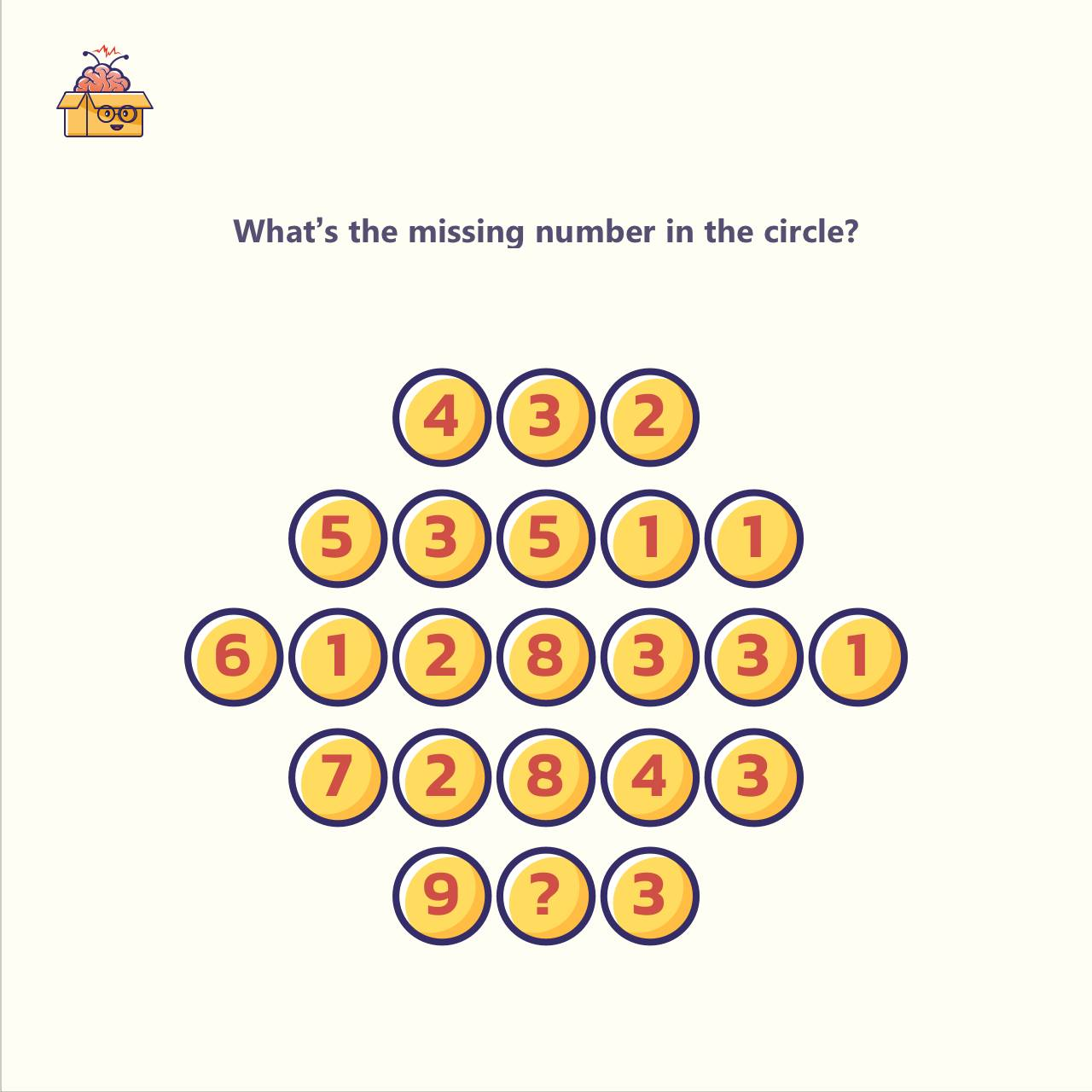## Brain Games, Brain Teasers, Math Puzzles

### If you can answer this, you are a certified genius. (Hint: You gotta think out of the box.)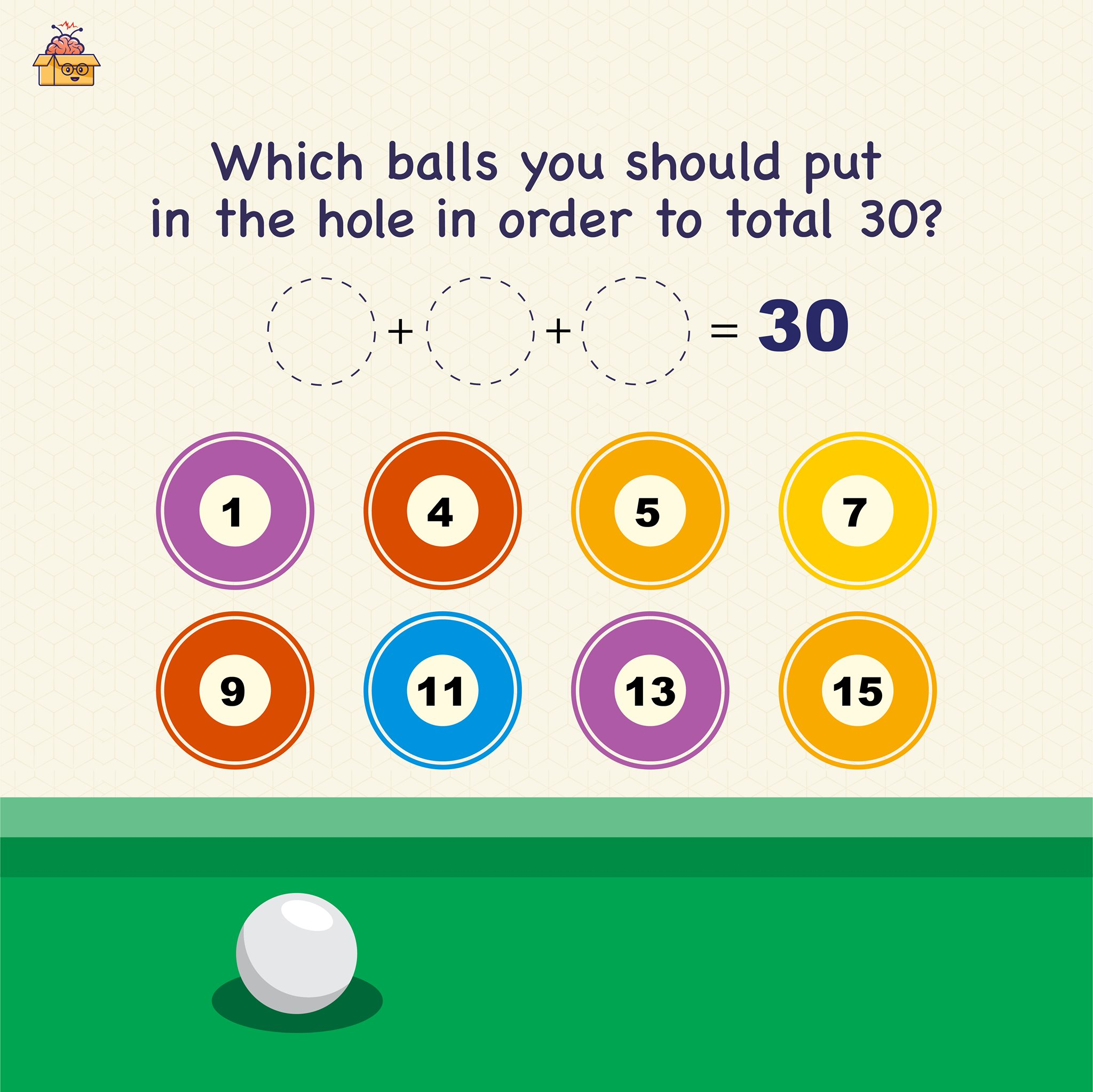## Logic Puzzles, Math Puzzles

### Here’s another classic puzzle that leaves most people scratching their heads. Do you know the answer? (Psst…Don’t Google it.)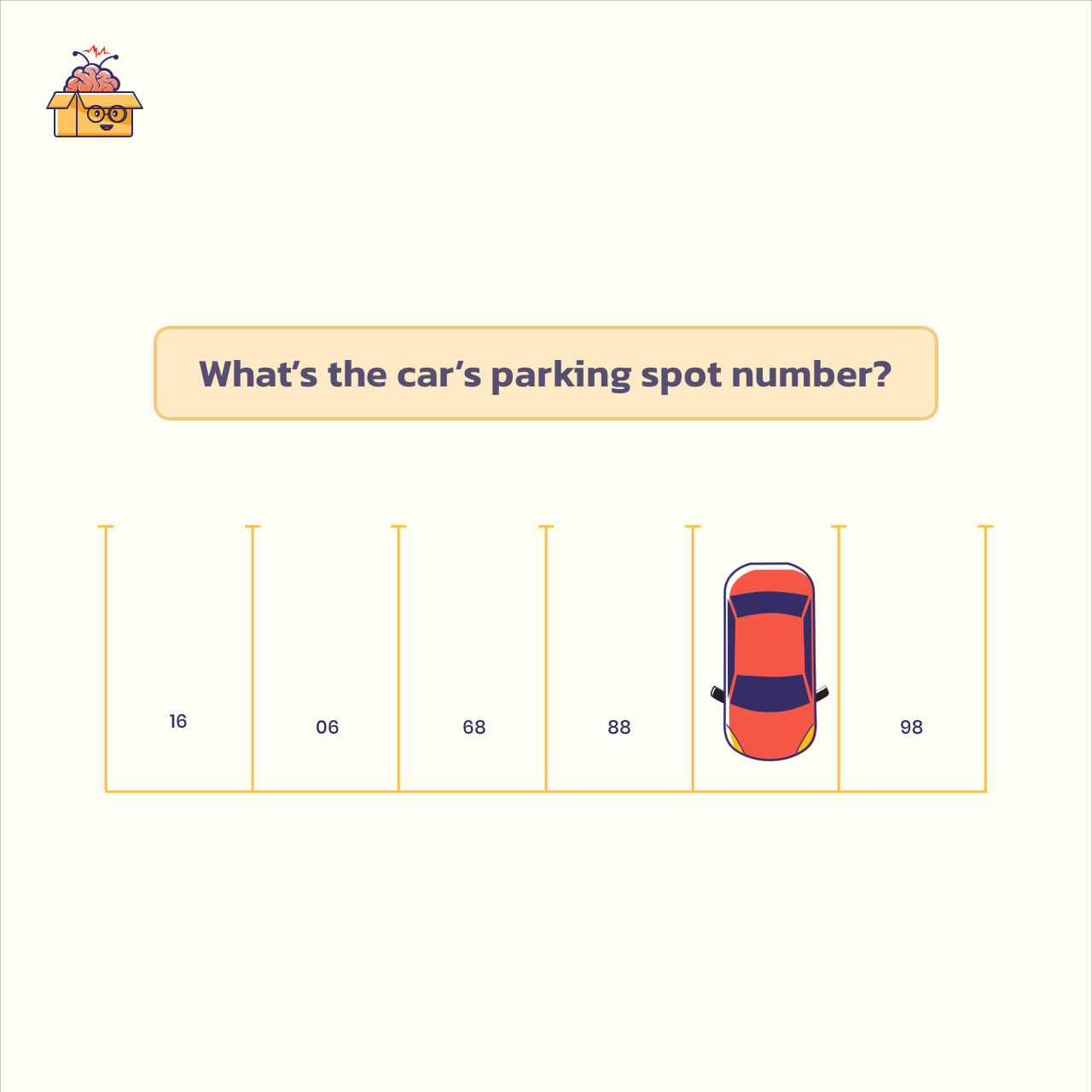## Brain Teasers, Logic Puzzles, Math Puzzles

### Here’s a Visual-Spatial Puzzle for you. Solving this kind of puzzle requires the ability to visualize things, visually understand the objects, and find the relation between them.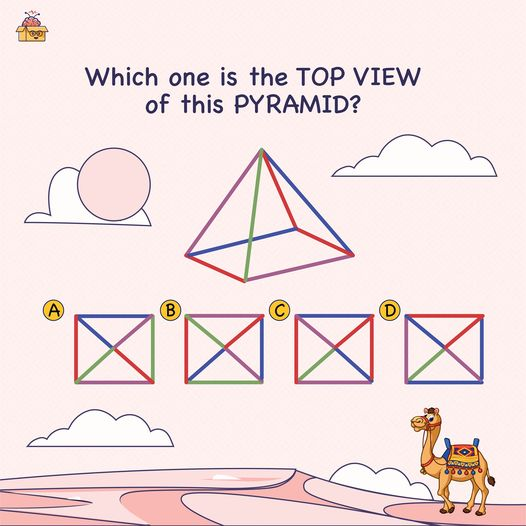## IQ Questions, Math Puzzles

### Alright, this one is complicated (and somewhat fruity). Can you solve this number puzzle? Share your answer in the comments.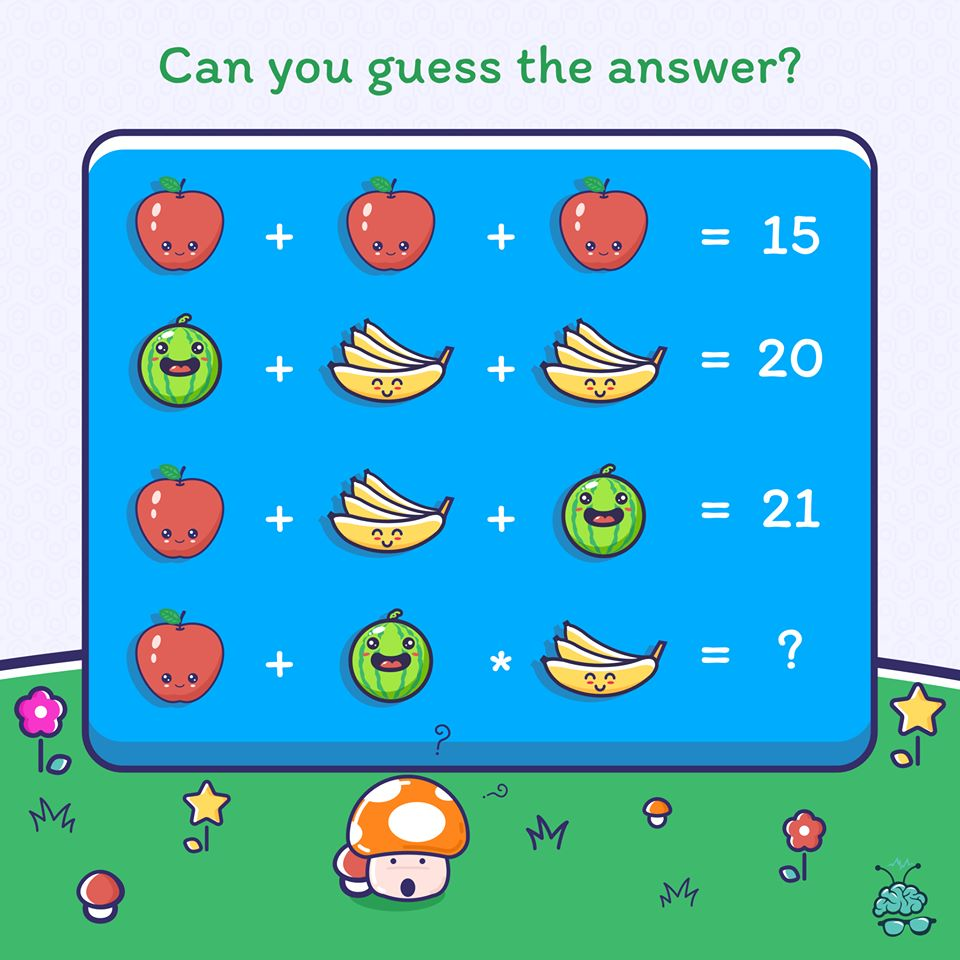## Math Puzzles

### Time for today’s question. Let’s see if you can solve this baffling math puzzle?